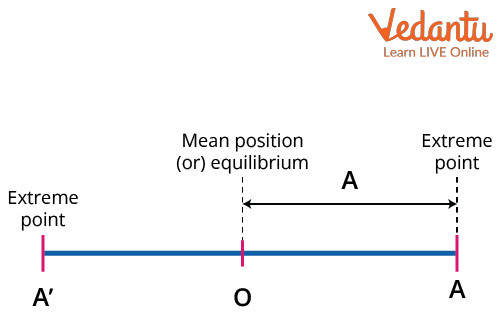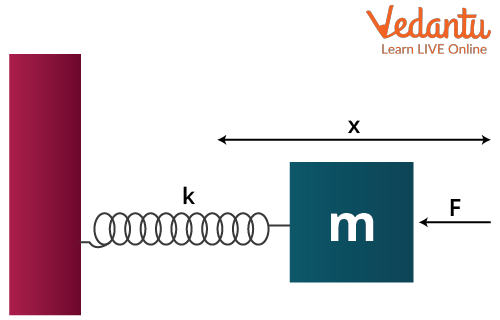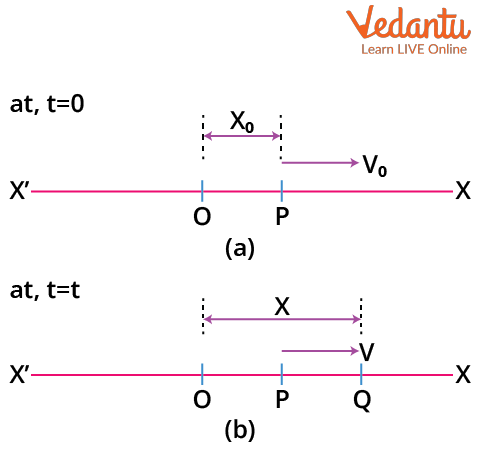Courses
Courses for Kids
Free study material
Free LIVE classes
More

# Time Period of Simple Harmonic Motion (SHM) with Formula and Examples for JEELIVE
Join Vedantu’s FREE Mastercalss

## What is Simple Harmonic Motion?

SHM is defined as a motion in which the magnitude of the restoring force is directly proportional to the body's displacement from its mean position.  It is always directed towards the mean position. Furthermore, all simple harmonic motions are periodic, but not all periodic motions are simple harmonic motions. The acceleration of a particle in simple harmonic motion is given by $a = – \omega^2 x$ . Here, $\omega$ is the angular velocity of the particle and  ‘x’ is the displacement of the spring from the equilibrium position.

## Terms Related to  SHM

### Mean Position

The point at which the particle's net force is zero.

### Mean Position Conditions

The force acting on the particle is inversely proportional to the displacement. As a result, this point of equilibrium will be stable.

### SHM Amplitude

It is the particle's maximum deviation from its mean position.SHM diagram

## Time Period of SHM

The time period of a particle executing SHM is defined as the shortest time taken to complete one oscillation or the minimum time after which the particle continues to repeat its motion. The period can be calculated by timing the duration of one complete oscillation where T gives the time period of the SHM formula. The two most common simple harmonic motion experiments are:

1. Pendulum: An oscillation with a period of a mass m attached to the end of a pendulum of length l. (T).

$T=2\pi\sqrt{\dfrac{l}{g}}$, where g represents gravitational acceleration.

2. Mass on a Spring: A mass m attached to a spring with a spring constant k will oscillate with a period (T).

$T=2\pi\sqrt{\dfrac{m}{k}}$ describes it.

It is worth noting that the period of a pendulum is independent of its mass, whereas the period of a mass on a spring is independent of the length of the spring. A simple harmonic oscillator's period is also independent of its amplitude.

3. Frequency of SHM: The frequency is defined as the number of oscillations per second. Angular frequency $\omega=2 \pi f=\dfrac{2 \pi}{ T}$ and frequency $=\dfrac{1}{T}$, where T is the time period of an oscillation.

This topic defines the amplitude and time period of simple harmonic motion.

## Simple Harmonic Motion Formula

Consider a spring with one end fixed. When no force is applied to the spring, it remains in its equilibrium position. When we pull the spring outward, it exerts a force that directs it towards the equilibrium position.

When we push the spring inward, a force is generated on it that is directed towards the equilibrium position. In both cases, the spring's force is directed towards the equilibrium position. This is also called the restoring force. Let's call the spring's force 'F' and the spring's displacement from its equilibrium position 'x'.SHM spring system diagram

The restoring force will be given by

F= – kx.

The negative sign denotes that the restoring force acts in the opposite direction.

Here, k is the force constant.

Consider a particle of mass (m) that is executing Simple Harmonic Motion along a path X’OX;  and the mean position is at point O. Let the speed of the particle be v0 when it is at position p (at a distance no from O)

At t = 0, the particle at P (moving towards the right)

At t = t, the particle is at Q (at a distance x from O)

With a velocity (v)A particle executing SHM

The restoring force at Q is given by

$\vec{F}=-K \vec{x}$

Here, K  is the positive constant

Also we have $\vec{F}=m \vec{a}$

Let a is the acceleration at Q

$m \vec{a}=-K \vec{x}$

This gives $\vec{d}=-\left(\dfrac{K}{m}\right) \vec{x}$

Put, $\dfrac{K}{m}=\omega^{2}$

So,

\begin{align} &\omega=\sqrt{\dfrac{K}{m}} \\ &\vec{a}=-\left(\dfrac{K}{m}\right) \vec{m}=-\omega^{2} \vec{x} \end{align}

As

$\vec{a}=\dfrac{d^{2} x}{d t^{2}}$

So,

$\dfrac{d^{2} \vec{x}}{d t^{2}}=-\omega^{2} \vec{x}$

This is the differential equation for linear simple harmonic motion

The time period of SHM is given as

$T=\dfrac{2 \pi}{\omega}$

We have already written for a spring system that

$\omega=\sqrt{\dfrac{K}{m}}$

This means that the time period can be written as

$T=2 \pi \sqrt{\dfrac{m}{K}}$

## Simple Harmonic Motion Examples

Simple harmonic motion is executed by any object that repeats its motion to and fro about a mean position over time.

Examples:

1. A basic pendulum.

2. System of mass springs

3. A steel ball rolling through a curved dish.

4. The swinging motion.

## Angular Simple Harmonic Motion

An angular oscillation can be produced by a body that is free to rotate about an axis. For example, a photo frame or a calendar hung from a nail on the wall. It produces angular oscillations when slightly pushed from its mean position and then released.

### Angular SHM Conditions for an Angular Oscillation

The body must feel a net torque that has been restored in nature. If the oscillation angle is small, the restoring torque will be proportional to the angular displacement. The expression of time period of angular SHM is as follows:

\begin{align} &\mathrm{T} \propto-\theta \\ &\mathrm{T}=-\mathrm{k} \theta \\ &\mathrm{T}=\mathrm{l} \alpha \\ &\alpha=-\mathrm{k} \theta \end{align}

So,

$I \dfrac{d^{2} \theta}{d t^{2}}=-K \theta$

$\dfrac{d^{2} \theta}{d t^{2}}=-\left(\dfrac{K}{I}\right) \theta=-\omega_{0}^{2} \theta$

$\dfrac{d^{2} \theta}{d t^{2}}=-\omega_{0}^{2} \theta=0$

This is the differential equation of an angular simple harmonic motion.

Now, we have that

\begin{align} &\omega^{2}=\dfrac{K}{I} \\ &\omega=\sqrt{\dfrac{K}{I}} \end{align}

We know that the time period T is given as

$T=\dfrac{2 \pi}{\omega}$

So, the time period for the angular SHM will be

$T=2 \pi \sqrt{\dfrac{I}{K}}$

## Solved Examples on Time Period of SHM

Example 1: The mass and the diameter of a planet are thrice the value of the Earth. The time period of a simple pendulum on the Earth is 10s. What will be its time period of oscillation of the same pendulum on the planet  ?

Solution:

Time period of oscillation means the time in which a simple pendulum will complete its one oscillation. Acceleration of the gravity is given by the formula :

$g=\dfrac{G M}{R^{2}}$

Let us suppose that gp and ge are the acceleration of gravity due to the planet and the Earth. Taking the ratio of the both, we have:

$\dfrac{g_{p}}{g_{e}}=\dfrac{M_{p}}{M_{e}}\left(\dfrac{R_{e}}{R_{p}}\right)^{2}$

as given

$\dfrac{M_{p}}{M_{e}}=3$

and

$\dfrac{R_{e}}{R_{p}}=\dfrac{1}{3}$

So,

$\dfrac{g_{p}}{g_{e}}=3\left(\dfrac{1}{3}\right)^{2}$

$\dfrac{g_{p}}{g_{e}}=\left(\dfrac{1}{3}\right)$

As T is inversely proportional to the square root of the acceleration due to gravity, so we will have:

\begin{align} &\dfrac{T_{p}}{T_{e}}=\sqrt{\dfrac{g_{p}}{g_{e}}} \\ &\dfrac{T_{p}}{T_{e}}=\sqrt{3} \\ &T_{n}=10 \sqrt{3} s \end{align}

Hence, the time period of oscillation of the same pendulum on the planet is $10 \sqrt{3} s$.

Example 2 : A simple pendulum oscillating in the air has time period T. The bob of the simple pendulum is now completely immersed in the non viscous liquid; given the density of the liquid is (1/8)th of the material of the bob. If the bob is inside the liquid all the time then what is the time period of oscillation of a simple pendulum of the bob in this liquid?

Solution:

The time period of oscillation for a simple pendulum is given according to the relation:

$T=2 \pi \sqrt{\dfrac{1}{\mathrm{~g}_{\mathrm{eff}}}}$

When we will immerse in the non viscous liquid, the acceleration due to gravity will become:

\begin{align} &g_{\text {eff }}=\left(g-\dfrac{g}{8}\right) \\ &g_{\text {eff }}=\left(\dfrac{7 g}{8}\right) \end{align}

Now, the ratio of the time period of the simple pendulum in the liquid to the time period in the air will be:

$\dfrac{T^{\prime}}{T}=\frac{2 \pi \sqrt{\dfrac{\mathrm{L}}{7 \mathrm{~g} / 8}}}{2 \pi \sqrt{\dfrac{\mathrm{L}}{\mathrm{g}}}}$

So,

\begin{align} &\dfrac{T^{\prime}}{T}=\sqrt{\dfrac{8}{7}} \\ &T^{\prime}=T \sqrt{\dfrac{8}{7}} \\ &T^{\prime}=T \sqrt{1.14} \\ &T^{\prime}=T(1.07) s \end{align}

Here, g represents gravitational acceleration, T’ represents the time period of the simple pendulum in the liquid and T represents the time period of the simple pendulum in the air.

## Conclusion

In short, the period of the simple harmonic motion can be determined by the time duration of one complete oscillation. We've learnt that simple harmonic motion (SHM) is a motion in which the magnitude of the restoring force is directly proportional to the body's displacement from its mean position. This restoring force is always directed towards the mean position. We also discussed the period of the particle's oscillation, its formula, examples and the various factors that help us understand the topic clearly.

Last updated date: 22nd Sep 2023
Total views: 132k
Views today: 2.32k

## FAQs on Time Period of Simple Harmonic Motion (SHM) with Formula and Examples for JEE

1. What is the use of simple harmonic motion?

The following are some real-world applications of SHM:

•  Clock

•  Instruments of music

•  Shock absorbers for automobiles

•  Jumping from a bungee

•  Scuba diving board

•  Hearing procedures

•  Metronome

Clock

A clock's time is kept by a pendulum or a vibrating quartz crystal. To ensure accurate time, the motion of the pendulum or quartz must be periodic.

Shock Absorbers for Automobiles

Springs attached to car wheels are required for a smooth ride for passengers. The wheels rise as the car passes over a bump in the road. If there are no shock absorbers in the car, the entire vehicle will be thrown up and down, making the passengers uncomfortable.

2. What are the linear SHM conditions?

The linear simple harmonic motion occurs when a particle moves back and forth along a straight line around a fixed point (called the equilibrium position). As an example, consider the spring-mass system. The restoring force or acceleration acting on the particle should always be proportional to the particle's displacement and directed towards the equilibrium position.

• The particles’ displacement from their equilibrium position

• The particle-acting restoring force

•  Acceleration of the particle